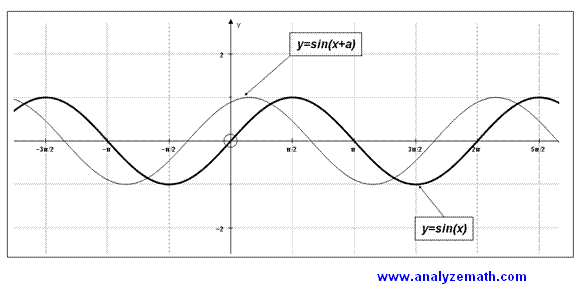# The Use of Graphing Software in the Mathematics Classroom (4)

 3.5 Mathematical Modeling and Problem Solving Problem: Find a function S that gives the number of squares needed to complete the pattern shown below for n rowsTask: Students use Autograph to plot the points (n ,S(n)) and find the best fit. As an extension to this work, students are asked to find S as a function of n analytically.Fig. 6: Best fit problem.. Students work in groups during this activity and use the best fit, which is another feature of Autograph, to find an equation whose graph passes through all four points. In a way this helps in introducing students to curve fitting. The second part of the activity, where students has to find an answer analytically, may be given as homework. 3.6 Explore the Concept of Periodicity Task: Students find the smallest positive value of the constant a, starting from a = 0, so that the graphs of sin x and sin (x + a) are the same.Fig. 7: Periodicity of sine function. Students worked individually on this simple activity which helped them understand the meaning of the period graphically. 1, 2, 3, 4, 5,

SEARCH THIS SITE

Custom Search